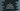# Write a C program to calculate profit or loss### C program to calculate profit or loss :

In this tutorial, we will learn how to find the profit or loss . We will get the cost price and selling price from the user and store it in different variables. And next, we will check if the user had made a profit or loss by finding the difference between cost price and selling price : selling price - cost price. If the value is more than 0, means _selling price _ is more than cost price , so the user has made a profit. Else, loss. We will also print out the value of the loss or profit.

### C program :

``````#include<stdio.h>

int main()
{
int cost_price,sell_price,result;

printf("Enter cost price : \n");
scanf("%d",&cost_price);

printf("Enter selling price : \n");
scanf("%d",&sell_price);

result = sell_price - cost_price;

if(result > 0){
printf("Profit : %d\n",result);
}else{
printf("Loss : %d\n",-result);
}

return 0;
}
``````

### Sample Output :

``````Enter cost price :
100
Enter selling price :
200
Profit : 100

Enter cost price :
300
Enter selling price :
100
Loss : 200
``````